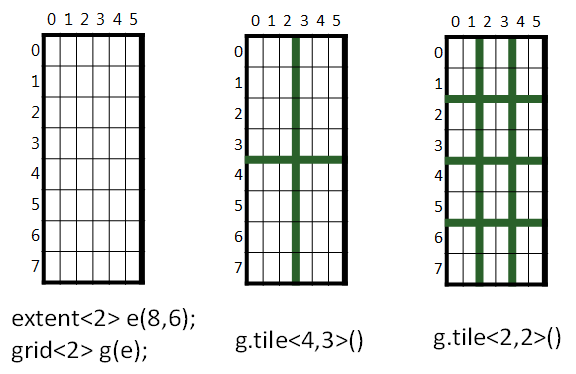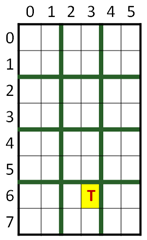## Scheduling thread tiles with C++ AMP

Sat, September 10, 2011, 08:30 PM under GPGPU | ParallelComputing

This post assumes you are totally comfortable with, what some of us call, the simple model of C++ AMP, i.e. you could write your own matrix multiplication. We are now ready to explore the tiled model, which builds on top of the non-tiled one.

#### Tiling the extent

We know that when we pass an extent to the parallel_for_each call, it determines the number of threads to schedule and their index values (including dimensionality). For the single-, two-, and three- dimensional cases you can go a step further and subdivide the threads into what we call tiles of threads (others may call them thread groups).

So here is a single-dimensional example:

```  extent<1> e(20);   // 20 units in a single dimension with indices from 0-19
tiled_extent<4> te = e.tile<4>();```

…on the 2nd line we subdivided the single-dimensional space into 5 single-dimensional tiles each having 4 elements, and we captured that result in a concurrency::tiled_extent (a new class in amp.h).

Let's move on swiftly to another example, in pictures, this time 2-dimensional:So we start on the left with a 2-dimensional extent which has 8*6=48 threads. We then have two different examples of tiling. In the first case, in the middle, we subdivide the 48 threads into tiles where each has 4*3=12 threads, hence we have 2*2=4 tiles. In the second example, on the right, we subdivide the original input into tiles where each has 2*2=4 threads, hence we have 4*3=12 tiles. Notice how you can play with the tile size and achieve different number of tiles. The numbers you pick must be such that the original total number of threads (in our example 48), remains the same, and every tile must have the same size.

Of course, you still have no clue why you would do that, but stick with me. First, we should see how we can use this tiled_extent, since the parallel_for_each function that we know expects a extent.

#### Tiled parallel_for_each and tiled_index

It turns out that we have additional overloads of parallel_for_each that accept a tiled_extent instead of a extent. However, those overloads, also expect that the lambda you pass in accepts a concurrency::tiled_index (new in amp.h), not an index<N>. So how is a tiled_index different to an index?

A tiled_index object, can have only 1 or 2 or 3 dimensions (matching exactly the tiled_extent), and consists of 4 index objects that are accessible via properties: global, local, tile_origin, and tile. The global index is the same as the index we know and love: the global thread ID. The local index is the local thread ID within the tile. The tile_origin index returns the global index of the thread that is at position 0,0 of this tile, and the tile index is the position of the tile in relation to the overall grid. Confused? Here is an example accompanied by a picture that hopefully clarifies things:```  array_view<int, 2> data(8, 6, p_my_data);
parallel_for_each(data.extent.tile<2,2>(), [=](tiled_index<2,2> t_idx) restrict(amp) { ... });```

Given the code above and the picture on the right, what are the values of each of the 4 index objects that the t_idx variables exposes, when the lambda is executed by T (highlighted in the picture on the right)?

If you can't work it out yourselves, the solution follows:

• t_idx.global       = index<2> (6,3)
• t_idx.local          = index<2> (0,1)
• t_idx.tile_origin = index<2> (6,2)
• t_idx.tile             = index<2> (3,1)

Don't move on until you are comfortable with this… the picture really helps, so use it.

#### Tiled Matrix Multiplication Example – part 1

Let's paste here the C++ AMP matrix multiplication example, bolding the lines we are going to change (can you guess what the changes will be?)

```01:  void MatrixMultiplyTiled_Part1(vector<float>& vC,
const vector<float>& vA,
const vector<float>& vB, int M, int N, int W)
02:  {
03:
04:    array_view<const float,2> a(M, W, vA);
05:    array_view<const float,2> b(W, N, vB);
06:    array_view<float,2> c(M, N, vC); c.discard_data();

07:    parallel_for_each(c.extent,
08:    [=](index<2> idx) restrict(amp) {
09:
10:      int row = idx; int col = idx;
11:      float sum = 0.0f;
12:      for(int i = 0; i < W; i++)
13:        sum += a(row, i) * b(i, col);
14:      c[idx] = sum;
15:    });
16:  }```

To turn this into a tiled example, first we need to decide our tile size. Let's say we want each tile to be 16*16 (which assumes that we'll have at least 256 threads to process, and that c.extent.size() is divisible by 256, and moreover that c.extent and c.extent are divisible by 16). So we insert at line 03 the tile size (which must be a compile time constant).

`03: static const int TS = 16;`

...then we need to tile the extent to have tiles where each one has 16*16 threads, so we change line 07 to be as follows

`07: parallel_for_each(c.extent.tile<TS,TS>(), `

...that means that our index now has to be a tiled_index with the same characteristics as the tiled_extent, so we change line 08

`08: [=](tiled_index<TS, TS> t_idx) restrict(amp) {`

...which means, without changing our core algorithm, we need to be using the global index that the tiled_index gives us access to, so we insert line 09 as follows

`09: index<2> idx = t_idx.global;`

...and now this code just works and it is tiled!

#### Closing thoughts on part 1

The process we followed just shows the mechanical transformation that can take place from the simple model to the tiled model (think of this as step 1). In fact, when we wrote the matrix multiplication example originally, the compiler was doing this mechanical transformation under the covers for us (and it has additional smarts to deal with the cases where the total number of threads scheduled is not divisible by the tile size). The point is that the thread scheduling is always tiled, even when you use the non-explicitly-tiled model.

But with this mechanical transformation, we haven't gained anything… Hint: our goal with explicitly using the tiled model is to gain even more performance.

In the next post, we'll evolve this further (beyond what the compiler can automatically do for us, in this first release), so you can see the full usage of the tiled model and its benefits…